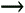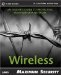# IV Collision

WEP uses a value known as an initialization vector, commonly called the IV. The RC4 algorithm uses this value to encrypt each packet with its own key. It does this by merging, or concatenating , the preshared password with the IV to create a new and exclusive "packet key" for each and every packet of information sent over the WLAN.

However, if the sending party uses an IV to encrypt the packet, the receiving party must also know this bit of information to decrypt the data. Because of the way WEP was implemented, this requirement turned an apparent strength into a weakness. Let's discuss this in more detail.

#### IV Explanation

WEP uses a three-byte IV for each packet of data transmitted over the WLAN. When the data is sent, the IV is prepended to the encrypted packet. This ensures the receiving party has all the information it needs to decrypt the data. However, if you take a closer look at the statistical nature of this process, you can quickly see a potential problem.

A byte is eight bits. Therefore, the total size of the IV is 24 bits (8 bits x3 bytes). If you calculate all the possible IVs, you would have a list of 2 24 possible keys. This number is derived from the fact that a bit can either be a 0 or a 1 (2), and there are a total of 24 bits ( 24 ). Although this might sound like a huge number (16,777,216), it is actually relatively small when associated with communication. The reason is the probability of repeats.

The IV is a random number. When most people tie the word random to a number like 16,777,216, their first assumption is that an attacker would have to wait for 16 million packets to be transferred before a repeat. This is false. In fact, as a result of the nature of randomness, one could expect to start seeing repeats, also known as collisions , after just 5,000 packet transmissions. Considering the average wireless device transmits a 1,500-byte packet, a collision could be expected with the transfer of just a 7 “10MB file. (For example, 5,000 packets x1500 bytes = 7,000,000 bytes [7MB].)

We will now illustrate this potential weakness. Our example assumes a hacker is about to send an email message packed with the character "2" repeated over and over. Although to the casual observer this might seem like an odd message, it helps the hacker crack WEP in his test lab.

#### Data Capture

The hacker first prepares to sniff the WLAN as the predictable data is transferred. As you will learn later in the book, this is a very simple task that anyone with a computer and wireless network card can do. In addition to sniffing during the expected data transmission, the hacker would have to maintain a listening status until he captured a matching IV. Again, as you learned before, this will probably take just a few minutes depending on the use of the WLAN.

At this point, the hacker has three pieces of information: the original data using IV, the ciphertext generated from the transmission of the original data with the IV, and the unknown ciphertext generated in another packet with the IV. The next step is to perform some bitwise calculations to decipher the unknown encrypted data.

#### Bitwise Comparisons

As you discovered in the first part of this chapter, you can deduce a keystream from ciphertext if you know the original data and ciphertext. This is represented by the following equation:

` Keystream = (Ciphertext) XOR (Plaintext) `
• Ciphertext 1 XOR Plaintext 2 = Ciphertext 2 XOR Plaintext 2

• Plaintext 1 XOR Plaintext 2 = Ciphertext 1 XOR Plaintext 2

• Plaintext 1&2 XOR Plaintext 1 = Plaintext 2

• Plaintext 1&2 XOR Plaintext 2 = Plaintext 1

• Ciphertext 1&2 XOR Ciphertext 1 = Ciphertext 2

• Ciphertext 1&2 XOR Ciphertext 2 = Ciphertext 1

Now, let's consider what we know. We have captured Ciphertext 1 and Ciphertext 2 . We also have Plaintext 1 ("2"). Knowing this, we can calculate Plaintext 2 ! Table 5.1 illustrates this concept:

` Ciphertext  1  XOR Ciphertext  2  = Plaintext  1  XOR Plaintext  2  `
##### Table 5.1. Using Captured Ciphertext Values in XOR Calculation to Deduce Plaintext 1XOR2

Keystream

Time

XOR Plaintext 2

Ciphertext 1

Ciphertext 2

Plaintext 1

1

00000010

XOR

01000011

=

01000001

2

00000010

XOR

01010010

=

01010000

3

00000100

XOR

01000110

=

01000010

4

00000010

XOR

01000000

=

01000010

5

00000010

XOR

01000100

=

01000110

6

00000100

XOR

01011010

=

01011110

7

00000010

XOR

01000001

=

01000011

8

00000010

XOR

01010111

=

01010101

9

00000100

XOR

01000110

=

01000010

From Table 5.1, we can see what the result of the calculation when XORing the two captured ciphertexts. Note that the resulting value is the XOR value of Plaintext 1 and Plaintext 2 . This is useless information unless you know one of the plaintext values, which can be used to produce the other plaintext value. Fortunately, in this situation the known plaintext ("2") can be XORed with the merged plaintext to produce the unknown plaintext. Table 5.2 shows how the following equation illustrates this process:

` Plaintext  1&2  XOR Plaintext  1  = Plaintext  2  `
##### Table 5.2. Using Known Plaintext 1 XOR with Merged Plaintext Value to Produce Unknown Plaintext 2

Keystream

Time

XOR Plaintext2

Plaintext1

Plaintext1

Plaintext2

1

01000001

XOR

00110001

=

01110000

2

01010000

XOR

00110001

=

01100001

3

01000010

XOR

00110001

=

01110011

4

01000010

XOR

00110001

=

01110011

5

01000110

XOR

00110001

=

01110111

6

01011110

XOR

00110001

=

01101111

7

01000011

XOR

00110001

=

01110010

8

01010101

XOR

00110001

=

01100100

9

01000010

XOR

00110001

=

01110011

So what valuable data did we capture? Table 5.3 shows the unknown captured value converted to ASCII characters .

` PlaintextASCII Conversion `

Plaintext2

ASCII

01110000

=

p

01100001

=

a

01110011

=

s

01110011

=

s

01110111

=

w

01101111

=

o

01110010

=

r

01100100

=

d

01110011

=

s

#### Discussion

Can you now see how dangerous collisions can be? Now suppose this data was your credit card number, or personal information about your health or credit history. As you can see, IV collisions are a serious issue that must accounted for in any WLAN.

This weakness is not so much the fault of WEP itself. It is instead a result of the limited number of IVs in the WEP process. If WEP used more IVs to keep track of and control the encryption process, the time between repeated values would be longer. Each added IV would drastically increase the amount of time between collisions, which in turn would affect the amount of time a hacker would have to spend collecting data in the hopes of capturing weak IVs.

Regardless of the benefits, the protocol designers wanted to ensure that they maximized the data flow and minimized the overhead. As a result, WEP was designed using the relatively small amount of data the IV requires in 802.11b/802.11a (3 bytes of 1,500 bytes). Ironically, with only a few more bytes devoted to security, WEP would have been much stronger, and most likely could have avoided many of the security issues surrounding it today.

For our example, we used actual values that would appear as a result of a data transmission. Do not be fooled into thinking your data is safe because of the massive amounts of information traveling across a network or WLAN. Hackers know the format, and often set up such programs to pull passwords right out of the rest of the data. As you will learn in Chapter 9, "Auditing Tools," sniffers can pick out passwords automatically and highlight them in the captured data.Maximum Wireless Security
ISBN: 0672324881
EAN: 2147483647
Year: 2002
Pages: 171

Similar book on Amazon